# Selina solutions for Concise Mathematics Class 8 ICSE chapter 18 - Constructions [Latest edition]

#### Chapters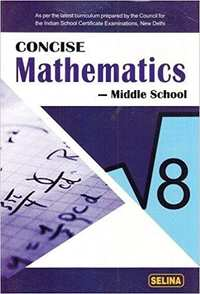## Chapter 18: Constructions

Exercise 18 (A)Exercise 18 (B)Exercise 18 (C)Exercise 18 (D)
Exercise 18 (A) [Page 202]

### Selina solutions for Concise Mathematics Class 8 ICSE Chapter 18 Constructions Exercise 18 (A) [Page 202]

Exercise 18 (A) | Q 1 | Page 202

Given below are the angles x and y.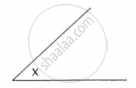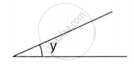Without measuring these angles, construct :

(i)

(ii)

(iii)

Exercise 18 (A) | Q 2 | Page 202

Given below are the angles x, y, and z.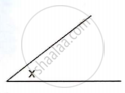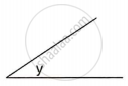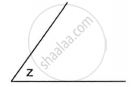Without measuring these angles construct :

(i) (ii) (iii)

Exercise 18 (A) | Q 3 | Page 202

Draw a line segment BC = 4 cm. Construct angle ABC = 60°.

Exercise 18 (A) | Q 4 | Page 202

Construct angle ABC = 45° in which BC = 5 cm and AB = 4.6 cm.

Exercise 18 (A) | Q 5 | Page 202

Construct angle ABC = 90°. Draw BP, the bisector of angle ABC. State, the measure of angle PBC.

Exercise 18 (A) | Q 6 | Page 202

Draw angle ABC of any suitable measure.

(i) Draw BP, the bisector of angle ABC.
(ii) Draw BR, the bisector of angle PBC and draw BQ, the bisector of angle ABP.
(iii) Are the angles ABQ, QBP, PBR and RBC equal?
(iv) Are the angles ABR and QBC equal?

Exercise 18 (B) [Page 204]

### Selina solutions for Concise Mathematics Class 8 ICSE Chapter 18 Constructions Exercise 18 (B) [Page 204]

Exercise 18 (B) | Q 1 | Page 204

Draw a line segment AB of length 5.3 cm. Using two different methods bisect AB.

Exercise 18 (B) | Q 2 | Page 204

Draw a line segment PQ = 4.8 cm. Construct the perpendicular bisector of PQ.

Exercise 18 (B) | Q 3 | Page 204

In each of the following, draw perpendicular through point P to the line segment AB :

(i)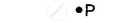(ii)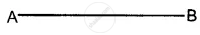(iii)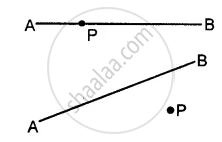Exercise 18 (B) | Q 4 | Page 204

Draw a line segment AB = 5.5 cm. Mark a point P, such that PA = 6 cm and PB = 4.8 cm. From point P, draw a perpendicular to AB.

Exercise 18 (B) | Q 5 | Page 204

Draw a line segment AB = 6.2 cm. Mark a point P in AB such that BP = 4 cm. Through point P draw perpendicular to AB.

Exercise 18 (C) [Page 205]

### Selina solutions for Concise Mathematics Class 8 ICSE Chapter 18 Constructions Exercise 18 (C) [Page 205]

Exercise 18 (C) | Q 1 | Page 205

Draw a line AB = 6 cm. Mark a point P anywhere outside the line AB. Through point P, construct a line parallel to AB.

Exercise 18 (C) | Q 2 | Page 205

Draw a line MN = 5.8 cm. Locate a point A which is 4.5 cm from M and 5 cm from N. Through A draw a line parallel to line MN.

Exercise 18 (C) | Q 3 | Page 205

Draw a straight line AB = 6.5 cm. Draw another line which is parallel to AB at a distance of 2.8 cm from it.

Exercise 18 (C) | Q 4 | Page 205

Construct an angle PQR = 80°. Draw a line parallel to PQ at a distance of 3 cm from it and another line parallel to QR at a distance of 3.5 cm from it. Mark the point of intersection of these parallel lines as A.

Exercise 18 (C) | Q 5 | Page 205

Draw an angle ABC = 60°. Draw the bisector of it. Also, draw a line parallel to BC a distance of 2.5 cm from it.
Let this parallel line meet AB at point P and angle bisector at point Q. Measure the length of BP and PQ. Is BP = PQ?

Exercise 18 (C) | Q 6 | Page 205

Construct an angle ABC = 90°. Locate a point P which is 2.5 cm from AB and 3.2 cm from BC.

Exercise 18 (D) [Pages 211 - 212]

### Selina solutions for Concise Mathematics Class 8 ICSE Chapter 18 Constructions Exercise 18 (D) [Pages 211 - 212]

Exercise 18 (D) | Q 1.1 | Page 211

AB = 4.3 cm, BC = 5.4, CD = 5 cm, DA = 4.8 cm and angle ABC = 75°.

Exercise 18 (D) | Q 1.2 | Page 211

AB = 6 cm, CD = 4.5 cm, BC = AD = 5 cm and ∠BCD = 60°.

Exercise 18 (D) | Q 1.3 | Page 211

AB = 8 cm, BC = 5.4 cm, AD = 6 cm, ∠A = 60° and ∠B = 75°.

Exercise 18 (D) | Q 1.4 | Page 211

AB = 5 cm, BC = 6.5 cm, CD =4.8 cm, ∠B = 75° and ∠C = 120°.

Exercise 18 (D) | Q 1.5 | Page 211

AB = 6 cm = AC, BC = 4 cm, CD = 5 cm and AD = 4.5 cm.

Exercise 18 (D) | Q 1.6 | Page 211

AB = AD = 5cm, BD = 7 cm and BC = DC = 5.5 cm

Exercise 18 (D) | Q 2.1 | Page 211

Construct a parallelogram ABCD, if :

AB = 3.6 cm, BC = 4.5 cm and ∠ABC = 120°.

Exercise 18 (D) | Q 2.2 | Page 211

Construct a parallelogram ABCD, if :

BC = 4.5 cm, CD = 5.2 cm and ∠ADC = 75°.

Exercise 18 (D) | Q 2.3 | Page 211

Construct a parallelogram ABCD, if :

AD = 4 cm, DC = 5 cm and diagonal BD = 7 cm.

Exercise 18 (D) | Q 2.4 | Page 211

Construct a parallelogram ABCD, if :

AB = 5.8 cm, AD = 4.6 cm and diagonal AC = 7.5 cm.

Exercise 18 (D) | Q 2.5 | Page 211

Construct a parallelogram ABCD, if :

diagonal AC = 6.4 cm, diagonal BD = 5.6 cm and angle between the diagonals is 75°.

Exercise 18 (D) | Q 2.6 | Page 211

Construct a parallelogram ABCD, if :

lengths of diagonals AC and BD are 6.3 cm and 7.0 cm respectively, and the angle between them is 45°.

Exercise 18 (D) | Q 2.7 | Page 211

Construct a parallelogram ABCD, if :

lengths of diagonals AC and BD are 5.4 cm and 6.7 cm respectively and the angle between them is 60°.

Exercise 18 (D) | Q 3.1 | Page 211

Construct a rectangle ABCD; if :

AB = 4.5 cm and BC = 5.5 cm.

Exercise 18 (D) | Q 3.2 | Page 211

Construct a rectangle ABCD; if :

BC = 6.1 cm and CD = 6.8 cm.

Exercise 18 (D) | Q 3.3 | Page 211

Construct a rectangle ABCD; if :

AB = 5.0 cm and diagonal AC = 6.7 cm.

Exercise 18 (D) | Q 3.4 | Page 211

Construct a rectangle ABCD; if :

AD = 4.8 cm and diagonal AC = 6.4 cm.

Exercise 18 (D) | Q 3.5 | Page 211

Construct a rectangle ABCD; if :

each diagonal is 6 cm and the angle between them is 45°.

Exercise 18 (D) | Q 3.6 | Page 211

Construct a rectangle ABCD; if :

each diagonal is 5.5 cm and the angle between them is 60°.

Exercise 18 (D) | Q 4.1 | Page 211

Construct a rhombus ABCD, if ;

AB = 4 cm and ∠B = 120°.

Exercise 18 (D) | Q 4.2 | Page 211

Construct a rhombus ABCD, if ;

BC = 4.7 cm and ∠B = 75°.

Exercise 18 (D) | Q 4.3 | Page 211

Construct a rhombus ABCD, if ;

CD = 5 cm and diagonal BD = 8.5 cm.

Exercise 18 (D) | Q 4.4 | Page 211

Construct a rhombus ABCD, if ;

BC = 4.8cm, and diagonal AC = 7cm.

Exercise 18 (D) | Q 4.5 | Page 211

Construct a rhombus ABCD, if ;

diagonal AC = 6 cm and diagonal BD = 5.8 cm.

Exercise 18 (D) | Q 4.6 | Page 211

Construct a rhombus ABCD, if ;

diagonal AC = 4.9 cm and diagonal BD = 6 cm.

Exercise 18 (D) | Q 4.7 | Page 211

Construct a rhombus ABCD, if ;

diagonal AC = 6.6 cm and diagonal BD = 5.3 cm.

Exercise 18 (D) | Q 5.1 | Page 211

Construct a square, if :

it's one side is 3.8 cm.

Exercise 18 (D) | Q 5.2 | Page 211

Construct a square, if :

its each side is 4.3 cm.

Exercise 18 (D) | Q 5.3 | Page 211

Construct a square, if :

one diagonal is 6.2 cm.

Exercise 18 (D) | Q 5.4 | Page 211

Construct a square, if :

each diagonal is 5.7 cm.

Exercise 18 (D) | Q 6 | Page 212

Construct a quadrilateral ABCD in which ; ∠A = 120°, ∠B = 60°, AB = 4 cm, BC = 4.5 cm and CD = 5 cm.

Exercise 18 (D) | Q 7 | Page 212

Construct a quadrilateral ABCD, such that AB = BC = CD = 4.4 cm, ∠B = 90° and ∠C = 120°.

Exercise 18 (D) | Q 8 | Page 212

Using ruler and compasses only, construct a parallelogram ABCD, in which : AB = 6 cm, AD = 3 cm and ∠DAB = 60°. In the same figure draw the bisector of angle DAB and let it meet DC at point P. Measure angle APB.

Exercise 18 (D) | Q 9 | Page 212

Draw a parallelogram ABCD, with AB = 6 cm, AD = 4.8 cm and ∠DAB = 45°. Draw the perpendicular bisector of side AD and let it meet AD at point P. Also, draw the diagonals AC and BD; and let them intersect at point O. Join O and P. Measure OP.

Exercise 18 (D) | Q 10 | Page 212

Using a ruler and compasses only, construct a rhombus whose diagonals are 8 cm and 6 cm. Measure the length of its one side.

## Chapter 18: Constructions

Exercise 18 (A)Exercise 18 (B)Exercise 18 (C)Exercise 18 (D)## Selina solutions for Concise Mathematics Class 8 ICSE chapter 18 - Constructions

Selina solutions for Concise Mathematics Class 8 ICSE chapter 18 (Constructions) include all questions with solution and detail explanation. This will clear students doubts about any question and improve application skills while preparing for board exams. The detailed, step-by-step solutions will help you understand the concepts better and clear your confusions, if any. Shaalaa.com has the CISCE Concise Mathematics Class 8 ICSE solutions in a manner that help students grasp basic concepts better and faster.

Further, we at Shaalaa.com provide such solutions so that students can prepare for written exams. Selina textbook solutions can be a core help for self-study and acts as a perfect self-help guidance for students.

Concepts covered in Concise Mathematics Class 8 ICSE chapter 18 Constructions are Introduction of Constructions, Construction of an Angle, To Construct an Angle Equal to Given Angle, To Draw the Bisector of a Given Angle, Construction of Angles of 60°,30°,90° and 45°, Construction of Bisector of a Line, Construction of Parallel Lines, Construction of Rhombus, Construction of Square, Construction of Parallelograms, Drawing the Perpendicular Bisector of a Line Segment, Construction of a Rectangle When Its Length and Breadth Are Given., Constructing a Quadrilateral, Concept of Reflection Symmetry.

Using Selina Class 8 solutions Constructions exercise by students are an easy way to prepare for the exams, as they involve solutions arranged chapter-wise also page wise. The questions involved in Selina Solutions are important questions that can be asked in the final exam. Maximum students of CISCE Class 8 prefer Selina Textbook Solutions to score more in exam.

Get the free view of chapter 18 Constructions Class 8 extra questions for Concise Mathematics Class 8 ICSE and can use Shaalaa.com to keep it handy for your exam preparation